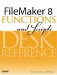# Ceiling()

Category: Number

 Syntax: Ceiling ( number )

Parameters:

numberAny expression that resolves to a numeric value.

Data type returned: Number

Description:

Returns number rounded up to the next integer.

One common use for the Ceiling function is finding out how many pages will be required to print x items if y items fit on a page. The formula for this is Ceiling ( x / y ). For instance, if you have 16 items, and 5 can print per page, you would need Ceiling ( 16/5 ) = Ceiling ( 3.2 ) = 4 pages.

Examples:

``` Ceiling ( 1.05 ) = 2

Ceiling ( -4.6 ) = -4

Ceiling ( 3 ) = 3
```FileMaker 8 Functions and Scripts Desk Reference
ISBN: 0789735113
EAN: 2147483647
Year: 2004
Pages: 352